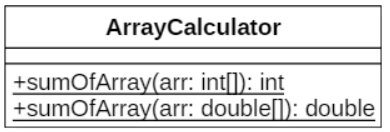# Bài tập nạp chồng phương thức trong Java | Codelearn

•TuanLQ7
• Easy
• 100 Points

Create an `ArrayCalculator` class in the file `ArrayCalculator.java` with the following information:Explanation

• `ArrayCalculator` is a class without attributes.
• `sumOfArray(arr: int[])` is a `static` method of which the input is an array of integers and it returns the sum of this array.
• `sumOfArray(arr: double[])` is a `static` method of which the input is an array of double numbers and it returns the sum of this array.

A program to test the above `Array``Calculator` class:

``````class Entry {
public static void main(String[] args) {
int[] arr1 = new int[] {3, 4, 2};
double[] arr2 = new double[] {1.3, 4.2, 6.7};
System.out.println(ArrayCalculator.sumOfArray(arr1));
System.out.println(ArrayCalculator.sumOfArray(arr2));
}
}
``````

When the above code is compiled and executed, it produces the following result:

``````9
12.2
``````

### Instruction

Code sample:

``````public class ArrayCalculator {
public static int sumOfArray(int arr[]) {
int sum = 0;
for (int i = 0; i < arr.length; i++) {
sum += arr[i];
}
return sum;
}

public static double sumOfArray(double arr[]) {
double sum = 0;
for (int i = 0; i < arr.length; i++) {
sum += arr[i];
}
return sum;
}
}``````